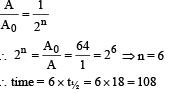Courses

# Single Correct MCQ Of Modern Physics, Past Year Questions JEE Advance, Class 12, Physics

## 60 Questions MCQ Test Class 12 Physics 35 Years JEE Mains &Advance Past year Paper | Single Correct MCQ Of Modern Physics, Past Year Questions JEE Advance, Class 12, Physics

Description
This mock test of Single Correct MCQ Of Modern Physics, Past Year Questions JEE Advance, Class 12, Physics for JEE helps you for every JEE entrance exam. This contains 60 Multiple Choice Questions for JEE Single Correct MCQ Of Modern Physics, Past Year Questions JEE Advance, Class 12, Physics (mcq) to study with solutions a complete question bank. The solved questions answers in this Single Correct MCQ Of Modern Physics, Past Year Questions JEE Advance, Class 12, Physics quiz give you a good mix of easy questions and tough questions. JEE students definitely take this Single Correct MCQ Of Modern Physics, Past Year Questions JEE Advance, Class 12, Physics exercise for a better result in the exam. You can find other Single Correct MCQ Of Modern Physics, Past Year Questions JEE Advance, Class 12, Physics extra questions, long questions & short questions for JEE on EduRev as well by searching above.
QUESTION: 1

### The plate resistance of a triode is 3 × 103 ohms and its mutual conductance is 1.5 × 10–3 amp/volt. The amplification factor of the triode is

Solution:

KEY CONCEPT : We know that µ = gm × r0
where µ = amplification factor,
gm = mutual conductance
r0 = plate resistance
∴ µ = 3 × 103 × 1.5 × 10–3 = 4.5

QUESTION: 2

### The half life of radioactive Radon is 3.8 days. The time at the end of which 1/20 th of the radon sample will remainundecayed is (given log10 e = 0.4343)

Solution:

t1/2 = 3.8 day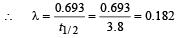If the initial number of atom is a = A0 then after time t the number of atoms is a/20 = A. We have to find t.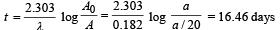QUESTION: 3

### An alpha particle of energy 5 MeV is scattered through 180° by a fixed uranium nucleus. The distance of closest approach is of the order of

Solution:

One point charge is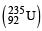uranium nucleus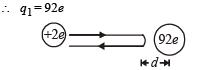The other point charge is α particle  ∴ q= + 2e
Here the loss in K.E. = Gain in P.E. (till α-particle reaches the distance d)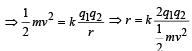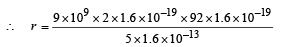= 529.92 × 10–16 m
= 529.92 × 10–14 cm
= 5.2992 × 10–12 cm

QUESTION: 4

Beta rays emitted by a radioactive material are

Solution:

β-particles are charged particles emitted by the nucleus.

QUESTION: 5

If elements with principal quantum number n > 4 were not allowed in nature, the  number of possible elements would be

Solution:

KEY CONCEPT : The maximum number of electrons in an orbit is 2n2. n > 4 is not allowed.
Therefore the number of maximum electron that can be in first four orbits are 2 (1)2 + 2 (2)2 + 2 (3)2 + 2 (4)2
= 2 + 8 + 18 + 32 = 60
Therefore, possible element are 60.

QUESTION: 6

Consider the spectral line resulting from the  transition n = 2 → n = 1 in the atoms and ions given below. The shortest wavelength is produced by

Solution:

We know that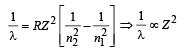λ is shortest when 1/λ is largest i.e., when Z has a highervalue. Z is highest for lithium.

QUESTION: 7

The equation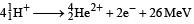represents

Solution: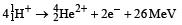QUESTION: 8

Fast neutrons can easily be slowed down by

Solution:

Fast neutrons can be easily slowed down by passing them through water.

QUESTION: 9

Consider α particles, β particles and γ - rays, each having an energy of 0.5 MeV. In increasing order of penetrating powers, the radiations are:

Solution:

Note : The penetrating power is dependent on velocity.
For a given energy, the velocity of γ radiation is highest and α-particle is least.

QUESTION: 10

An energy of 24.6 eV is required to remove one of the electrons from a neutral helium atom. The energy in (eV) required to remove both the electrons from a neutral helium atom is

Solution:

When one e is removed from neutral helium atom, it becomes a one e species.
For one e species we know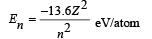For helium ion, Z = 2 and for first orbit n = 1.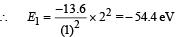∴ Energy required to remove this e  = + 54.4 eV

∴ Total energy required = 54.4 + 24.6 = 79 eV

QUESTION: 11

A radioactive material decays by simultaneous emission of two particles with respective half-lives 1620 and 810 years.
The time, in years, after which one-fourth of the material remains is

Solution: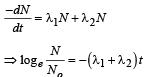when No is initial number of atoms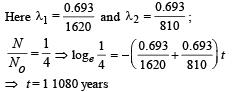QUESTION: 12

The probability of electrons to be found in the conduction band of an intrinsic semiconductor at a finite temperature

Solution:

KEY CONCEPT : For a semi conductor n = n0e–Eg/kT where n0 = no. of free electrons at absolute zero, n = no. of free electrons at T kelvin, Eg = Energy gap, k = Boltzmann constant.
As Eg increases, n decreases exponentially.

QUESTION: 13

A full-wave rectifier circuit along with the out-put is shown in Figure. The contribution (s) from the diode 1 is (are)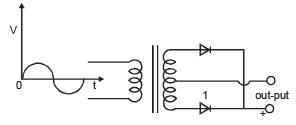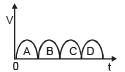Solution:

As shown in the fig. (i) during one half cycle the polarity of P and S are opposite such that diode (1) is reversed biased and hence non conducting.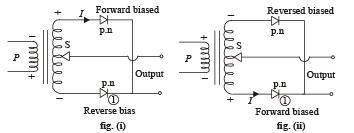During the other half cycle, diode (1) gets forward biased and is conducting. Thus diode (1) conducts in one half cycle and does not conduct in the other so the correct option is (b) (a and c.)

QUESTION: 14

As per Bohr model, the minimum energy (in eV) required to remove an electron from the ground state of doubly ionized Li atom (Z = 3) is

Solution:

KEY CONCEPT :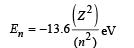Therefore, ground state energy of doubly ionized lithium atom (Z = 3, n = 1) will be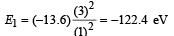∴ Ionization energy of an electron in ground state of doubly ionized lithium atom will be 122.4eV.

QUESTION: 15

The circuit shown in the figure contains two diodes each with a forward resistance of 50 ohms and with infinite backward resistance. If the battery voltage is 6V, the current through the 100 ohm resistance (in  amperes) is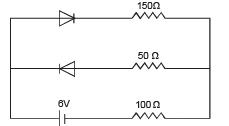Solution:

In the circuit, diode D1 is forward biased, while D2 is reverse biased. Therefore, current i (through D1 and 100Ω resistance) will be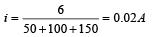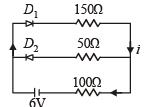Here, 50Ω is the resistance of D1 in forward biasing.

QUESTION: 16

Which of the following statements is not true?

Solution:

In n-type semiconductors, electrons are the majority charge carriers.

QUESTION: 17

The maximum kinetic energy of photoelectrons emitted from a surface when photons of energy 6 eV fall on it is 4 eV. The stopping potential, in volt, is

Solution:

Stopping potential is the negative potential applied to stop the electrons having maximum kinetic energy.
Therefore, stopping potential will be 4 volt.

QUESTION: 18

In hydrogen spectrum the wavelength of Hα line is  656 nm, whereas in the spectrum  of a distant galaxy, Hα line wavelength is 706 nm. Estimated speed of the galaxy with respect to earth is,

Solution:

KEY CONCEPT : According to Doppler’s effect of light, the wavelength shift is given by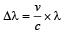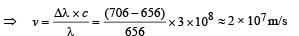QUESTION: 19

A particle of mass M at rest decays into two particles of masses m1 and m2, having non-zero velocities. The ratio of the de Broglie wavelengths of the particles, λ12, is

Solution:

Applying conservation of linear momentum, Initial momentum = Final momentum

0 = m1v1 – m2v2 ⇒ m1v1 = m2v2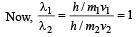QUESTION: 20

Which of the following is a correct statement?

Solution:

Beta rays are same as cathode rays as both are stream of electrons.

QUESTION: 21

Order of magnitude of density of uranium nucleus is, [mp = 1.67 × 10–27kg]

Solution:

Nuclear density of an atom of mass number A,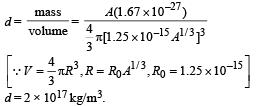QUESTION: 22

22Ne nucleus, after absorbing energy, decays into two aparticles and an unknown nucleus. The unknown nucleus is

Solution: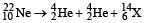The new element X has atomic number 6. Therefore, it is carbon atom.

QUESTION: 23

Binding energy per nucleon vs mass number curve for nuclei is shown in the Figure. W, X, Y and Z are four nuclei indicated on the curve. The process that would release energy is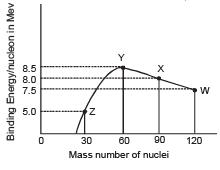Solution:

KEY CONCEPT : Energy is released when stability increases. This will happen when binding energy per nucleon increases.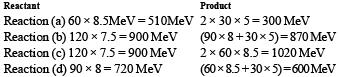QUESTION: 24

Imagine an atom made up of a proton and a hypothetical particle of double the mass of the electron but having the same charge as the electron. Apply the Bohr atom model and consider all possible transitions of this hypothetical particle to the first excited level. The longest wavelength photon that will be emitted has wavelength λ (given in terms of the Rydberg constant R for the hydrogen atom) equal to

Solution:

KEY CONCEPT :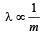For ordinary hydrogen atom, longest wavelength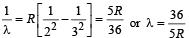With hypothetical particle, required wavelength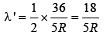QUESTION: 25

The electron in a hydrogen atom makes a transition from an excited state to the ground state. Which of the following statements is true ?

Solution:

NOTE : As the electron comes nearer to the nucleus the potential energy decreases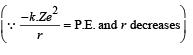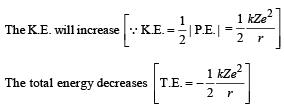QUESTION: 26

Two radioactive materials X1 and X2 have decay constants 10λ and λ respectively. If initially they have the same number of nuclei, then the ratio of the number of nuclei of X1 to that of X2 will be 1/e after a time

Solution: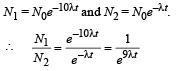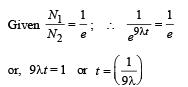QUESTION: 27

Electrons with energy 80 keV are incident on the tungsten target of an X-ray tube. K-shell electrons of tungsten have 72.5 keV energy. X-rays emitted by the tube contain only

Solution:

KEY CONCEPT :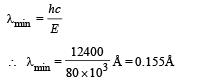Energy of incident electrons is greater than the ionization energy of electrons in K-shell, the K-shell electrons will be knocked off. Hence, characteristic X-ray spectrum will be obtained.

QUESTION: 28

The electron emitted in beta radiation originates from

Solution:

Note : In a nucleus neutron converts into proton as follows n → p+ + e–1 Thus, decay of neutron is responsible for b-radiation origination

QUESTION: 29

The transition from the state n = 4 to n = 3 in a hydrogen-like atom results in ultraviolet radiation. Infrared radiation will be obtained in the transition

Solution:

For 2 to 1, 3 to 2 and 4 to 2 we get energy that  n = 4 to n = 3,

QUESTION: 30

The intensity of X-rays from a Coolidge tube is plotted against wavelength λ as shown in the figure. The minimum wavelength found is λC and the wavelength of the Kα line is λK. As the accelerating voltage is increased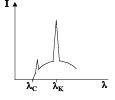Solution:

KEY CONCEPT :

In case of Coolidge tube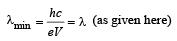Thus the cut off wavelength is inversely proportional to accelerating voltage. As V increases, λc decreases. λk is the wavelength of K line which is a characteristic of an atom and does not depend on accelerating voltage of bombarding electron since λk always refers to a photon wavelength of transition of e from the target element from 2 → 1.
The above two facts lead to the conclusion that λk – λc increases as accelerating voltage is increased.

QUESTION: 31

A radioactive sample consists of two distinct species having equal number of atoms initially. The mean life time of one species is τ and that of the other is 5τ. The decay products in both cases are stable. A plot is made of the total number of radioactive nuclei as a function of time. Which of the following figures best represent the form of this plot?

Solution: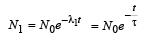....(i)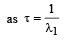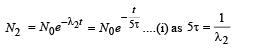Adding (i) and (ii) we get

N = N1 + N2 = N0 (e -t/τ+e-t/5τ

(a) is NOT the correct option as there is  a time τ for which N is constant which means for time τ there is no process of radioactivity which does not makes sense. (b) and (c) shows intermediate increase in the number of radioactive atom which is IMPOSSIBLE as N will only decrease exponentially.

QUESTION: 32

The potential difference applied to an X-ray tube is 5kV and the current through it is 3.2mA. Then the number of electrons striking the target per second is

Solution:

KEY CONCEPT :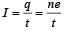No. of electrons striking the target per second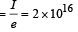QUESTION: 33

A Hydrogen atom and a Li++ ion are both in the second excited state. If ℓ H and ℓ Li are their respective electronic angular momenta, and EH and ELi their respective energies, then

Solution: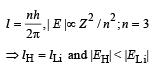QUESTION: 34

The half-life of 215At is 100 μ s. The time taken for the radioactivity of a sample of 215At to decay to 1/16th of  its initial value is

Solution:

A = A0 (1/2)n; n = number of half lives.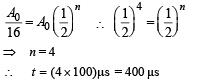QUESTION: 35

Which of the following processes represents a γ -decay ?

Solution:

In γ-decay, the atomic number and mass number do not change.

QUESTION: 36

The electric potential between a proton and an electron is given by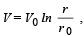where r0 is a constant. AssumingBohr’s model to be applicable, write variation of rn with n, n being the principal quantum number?

Solution:

Given potential energy between electron  and proton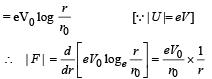But this force acts as centripetal force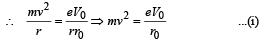By Bohr’s postulate, mvr  = nh/2π    ...(ii)

From (i) and (ii),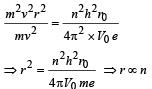QUESTION: 37

If the atom 100Fm257 follows the Bohr model and the radius of 100Fm257 is n times the Bohr radius, then find n.

Solution:

KEY CONCEPT : For an atom followig Bohr ’s model, the radius is given by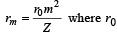= Bohr’s radius and m = orbit number.
For Fm, m = 5 (Fifth orbit in which the outermost electron is present)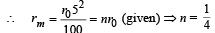QUESTION: 38

For uranium nucleus how does its mass vary with volume?

Solution:

KEY CONCEPT : We know that radius of the nucleus R = R0A1/3, where A is the mass number.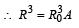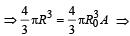Volume ∝ mass.

QUESTION: 39

A nucleus with mass number 220 initially at rest emits an α -particle. If the Q value of the reaction is 5.5 MeV, calculate the kinetic energy of the α -particle

Solution:

By conservation of momentum, p1 = p2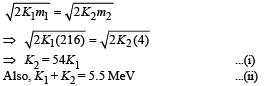Solve equation (i) and (ii)

QUESTION: 40

In a photoelectric experiment anode potential is plotted against plate current.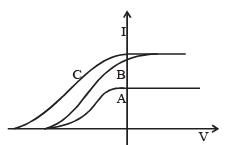Solution:

From the graph it is clear that A and B have the same stopping potential and therefore, the same frequency.
Also, B and C have the same intensity.

QUESTION: 41

A 280 days old radioactive substance shows an activity of 6000 dps, 140 days later its activity becomes 3000 dps.
What was its initial activity?

Solution:

In two half lives, the activity will remain 1/4 of its initial activity.

QUESTION: 42

A pr oton has kinetic ener gy E = 100 keV which is equal to that of a photon. The wavelength of photon is λ2 and that of proton is λ1. The ration of λ21 is proportional to

Solution:

For photon,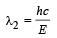For proton,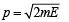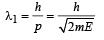...(ii)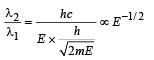QUESTION: 43

Kα wavelength emitted by an atom  of atomic number Z = 11 is λ. Find the atomic number for an atom that emits Kα radiation with wavelength 4λ.

Solution: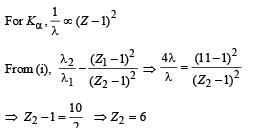QUESTION: 44

A photon collides with a stationary hydrogen atom in ground state inelastically. Energy of the colliding photon is 10.2 eV.
After a time interval of the order of micro second another photon collides with same hydrogen atom inelastically with an energy of 15 eV. What will be observed by the detector?

Solution:

Initially a photon of energy 10.2eV collides inelastically with a hydrogen atom in ground state. For hydrogen atom,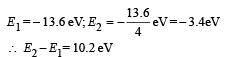The electron of hydrogen atom will jump to second orbit after absorbing the photon of energy 10.2 eV. The electron jumps back to its original state in less than microsecond and release a photon of energy 10.2 eV.
Another photon of energy 15 eV strikes the hydrogen atom inelastically. This energy is sufficient to knock out the electron from the atom as ionisation energy is 13.6 eV. The remaining energy of 1.4 eV is left with electron as its kinetic energy.

QUESTION: 45

A beam of electron is used in an YDSE experiment. The slit width is d. When the velocity of electron is increased, then

Solution:

Note : Since electron shows wave nature, it will show the phenomenon of interference.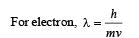When speed of electron increases, λ will decrease. The distance between two consecutive fringes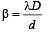As λ decreases, β also decrease.

QUESTION: 46

If a star can convert all the He nuclei completely into oxygen nuclei, the energy released per oxygen nuclei is  [Mass of He nucleus is 4.0026 amu and mass of Oxygen nucleus is 15.9994 amu]

Solution: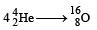B.E. = Δm × 931.5 MeV
= (4 × 4.0026 – 15.9994) × 931.5 = 10.24 MeV

QUESTION: 47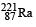is a radioactive substance having half life of 4 days. Find the probability that a nucleus undergoes decay after two half lives

Solution:

For a nucleus to disintegr ate in two half life, the probability is 3/4 as 75% of the nuclei will disintegrate in this time.

QUESTION: 48

In the options given below, let E denote the rest mass energy of a nucleus and n a neutron.The correct option is

Solution:

Iodine and Yttrium are medium sized nuclei and therefore, have more binding energy per nucleon as compared to Uranium which has a big nuclei and less B.E./nucleon.
In other words, Iodine and Yttrium are more stable and therefore possess less energy and less rest mass. Also when Uranium nuclei explodes, it will convert into I and Y nuclei having kinetic energies.

QUESTION: 49

The largest wavelength in the ultraviolet region of the hydrogen spectrum is 122 nm. The smallest wavelength in the infrared region of the hydrogen spectrum (to the nearest integer) is

Solution:

The smallest frequency and largest wavelength in ultraviolet region will be for transition of electron from orbit 2 to orbit 1.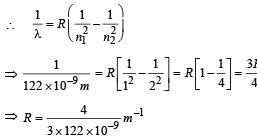The highest frequency and smallest wavelength for infrared region will be for transition of electron from ∞ to 3rd orbit.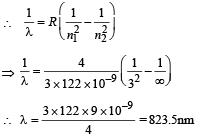QUESTION: 50

Electrons with de-Broglie wavelength λ fall on the target in an X-ray tube. The cut-off wavelength of the emitted X-rays is

Solution:

The cut off wavelength is given by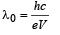...(i)

According to de Broglie equation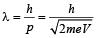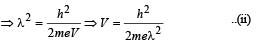From (i) and (ii),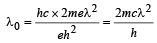QUESTION: 51

Which one of the following statements is WRONG in the context of X-rays generated from a X-ray tube?

Solution:

The continuous spectrum depends on the accelerating voltage. It has a definite minimum wavelength.
Greater the accelerating voltage for electrons, higher will be the kinetic energy it attains before striking the target, higher will be the frequency of X - rays and smaller will be the wavelength. The wavelength of continuous X - rays is independent of the atomic number of target material.

QUESTION: 52

A radioactive sample S1 having an activity 5µCi has twice the number of nuclei as another sample S2 which has an activity of 10 µCi. The half lives of S1 and S2 can be

Solution: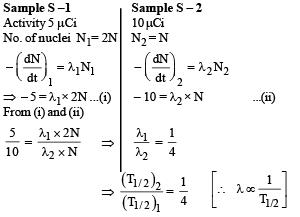QUESTION: 53

Photoelectric effect experiments are performed using three different metal plates p, q and r having work functions φp = 2.0 eV, φq = 2.5 eV and φr = 3.0 eV, respectively. A light beam containing wavelengths of 550 nm, 450 nm and 350  nm with equal intensities illuminates each of the plates. The correct I-V graph for the experiment is  [Take hc = 1240 eV nm]

Solution:

The energy possessed by photons of wavelen gth 550 nm is 1240/550 = 2.25eV
The energy possessed by photons of wavelength 450 nm is 1240/450 = 2.76eV

The energy possessed by photons of wavelength 350 nm is 1240/350 = 3.5.eV

For metal plate p :

φp = 2 eV.

Photons of wavelength 550 nm will not be able to eject electrons and therefore, the current is smaller than p.
The work function is greater than q therefore the stopping potential is lower in comparison to p.

For metal plate r :

φr = 3 eV

Only wavelength of 350 nm will be able to eject electrons and therefore, current is minimum. Also the stopping potential is least.

QUESTION: 54

The wavelength of the first spectral line in the Balmer series of hydrogen atom is 6561 A°. The wavelength of the second spectral line in the Balmer series of singly-ionized helium atom is

Solution: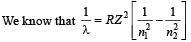The wave length of first spectral line in the Balmer series of hydrogen atom is 6561Å . Here n2 = 3 and n1 = 2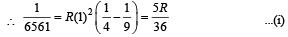For the second spectral line in the Balmer series of singly ionised helium ion n2 = 4 and n1 = 2 ; Z = 2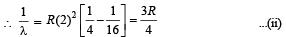Dividing equation (i) and equation (ii) we get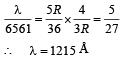QUESTION: 55

A pulse of light of duration 100 ns is absorbed completely by a small object initially at rest. Power of the pulse is 30 mW and the speed of light is 3×108 ms–1. The final momentum of the object is

Solution: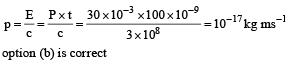QUESTION: 56

If λCu is the wavelength of Kα X-ray line of copper (atomic number 29) and λMo is the wavelength of the Kα X-ray line of molybdenum (atomic number 42), then the ratio λCuMo is close to

Solution: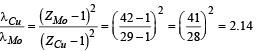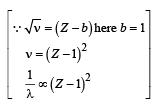QUESTION: 57

A metal surface is illuminat ed by light of two different wavelengths 248 nm and 310 nm. The maximum speeds of the photoelectrons corresponding to these wavelengths are u1 and u2, respectively. If the ratio u1 : u2 = 2 : 1 and hc = 1240 eV nm, the work function of the metal is nearly

Solution: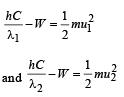Dividing the above two equations, we get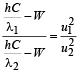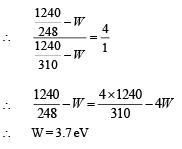QUESTION: 58

In a historical experiment to determine Planck's constant, a metal surface was irradiated with light of different wavelengths. The emitted photoelectron energies were measured by applying a stopping potential. The relevant data for the wavelength (λ) of incident light and the corresponding stopping potential (V0) are given below :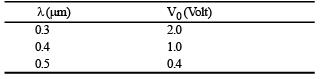Given that c = 3 × 108m s–1 and e = 1.6 × 10–19 C , Planck's constant (in units of J s) found from such an experiment is

Solution: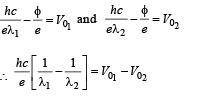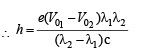From the first two values given in data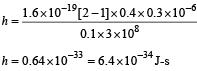Similarly if we calculate h  for the last two values of data  h = 6.4 × 10–34J-s

QUESTION: 59

The electrostatic energy of Z protons uniformly distributed throughout a spherical nucleus of radius R is given by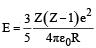The measured masses of the neutron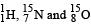are 1.008665 u, 1.007825 u, 15.000109 u and 15.003065 u, respectively. Given that the radii of both the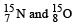nuclei are same, 1 u = 931.5 Me V/c2 (c is the speed of light) and e2/(4 πε0) = 1.44 MeV fm. Assuming  that the difference between the binding energies of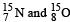is purely due to the electrostatic energy, the radius of either of the nuclei is (1 fm = 10–15 m)

Solution:

Binding energy of nitrogen atom
= [8 × 1.008665 + 7 × 1.007825 – 15.000109] × 931
Binding energy of oxygen atom
= [7 × 1.008665 + 8 × 1.007825 – 15.003065] × 931
∴ Difference = 0.0037960 × 931 MeV    ...(I)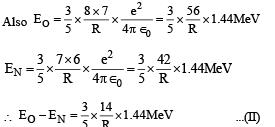From (i) & (ii)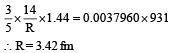QUESTION: 60

An accident in a nuclear labora tory resulted in deposition of a certain amount of radioactive material of half-life 18 days inside the laboratory. Tests revealed that the radiation was 64 times more than the permissible level required for safe operation of the laboratory. What is the minimum number of days after which  the laboratory can be considered safe for use?

Solution: